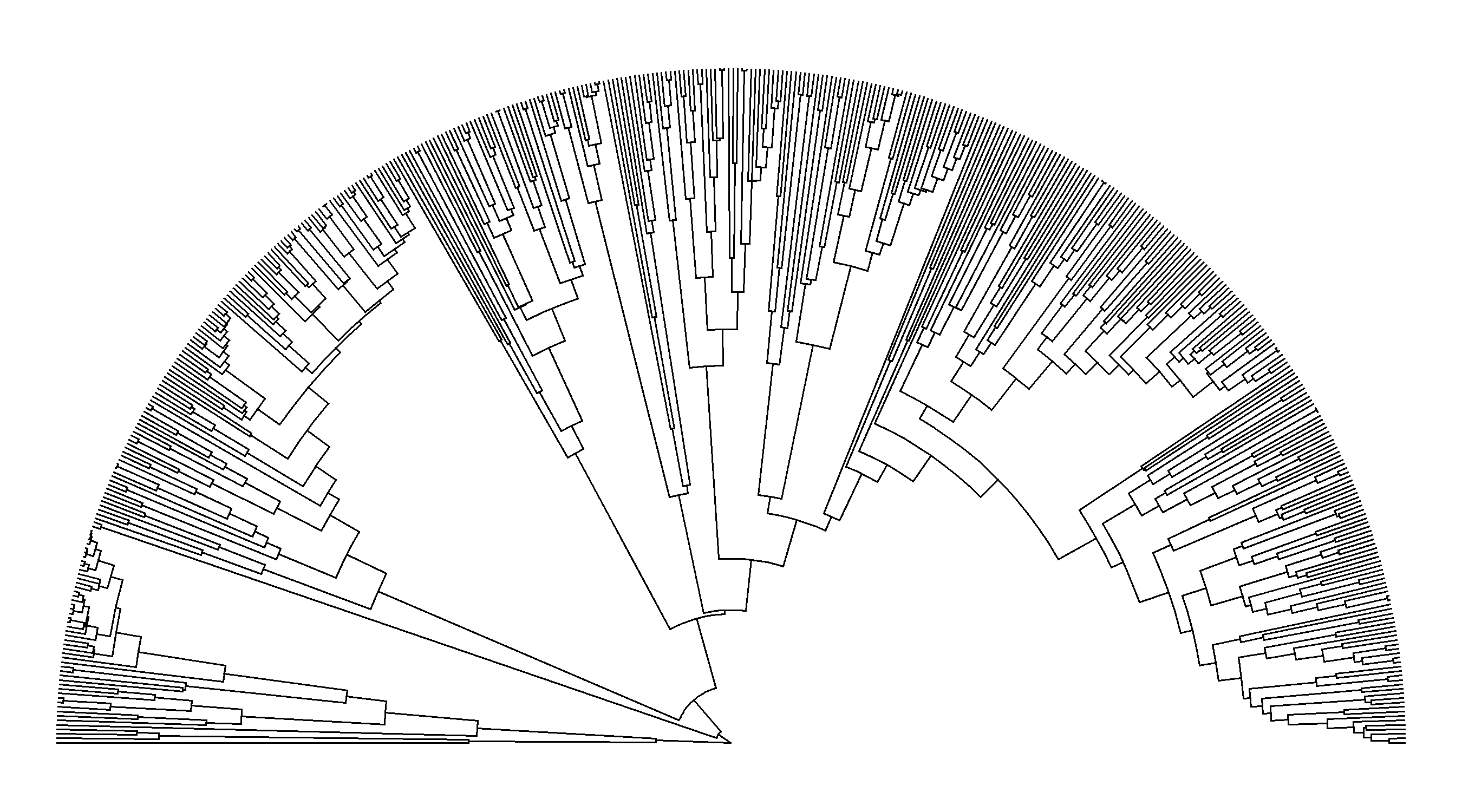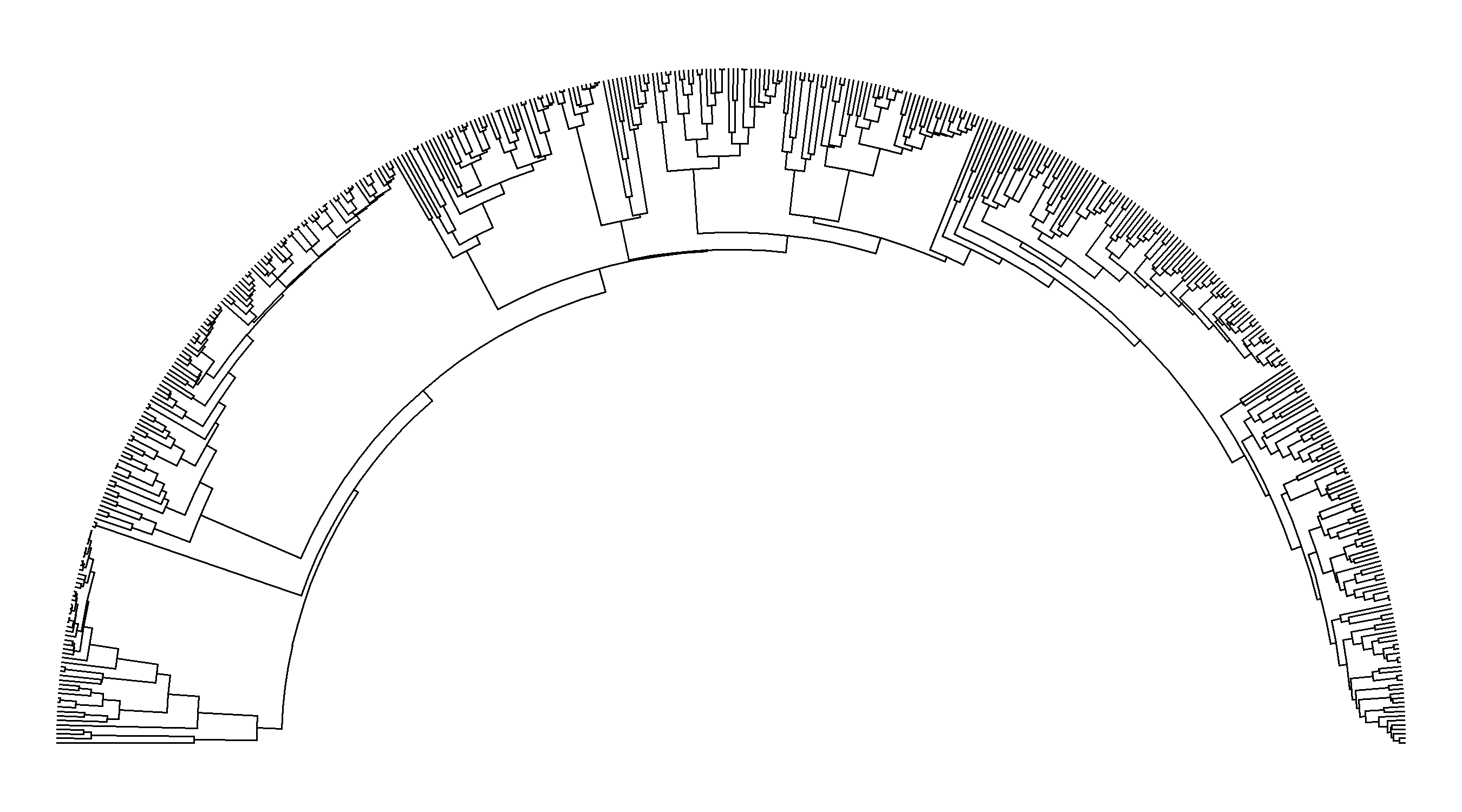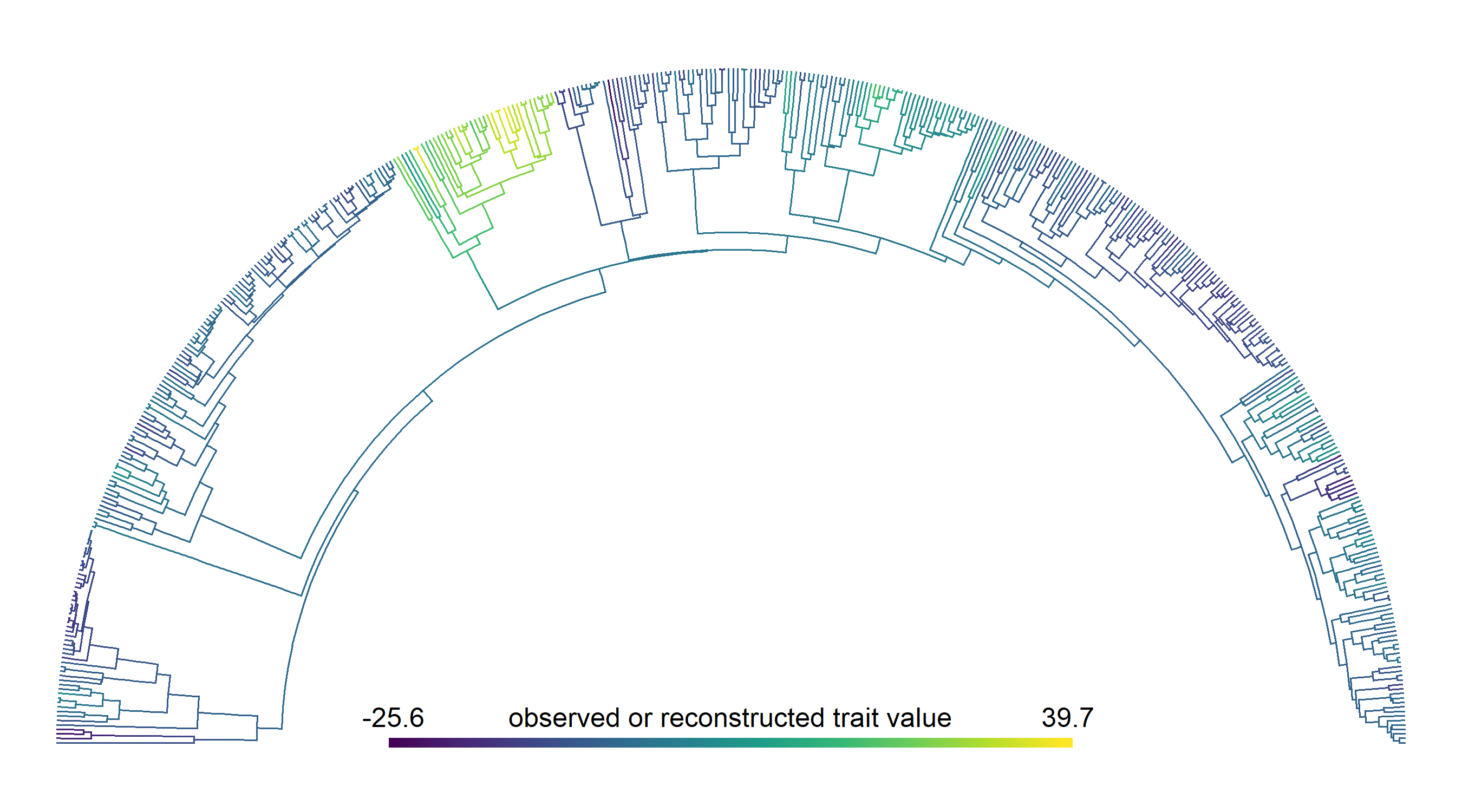## Friday, April 28, 2023

### Creating an "arc" style tree in R using phytools

I’ve been seeing quite a few phylogenies that one might best describe as “arc trees” – fan style trees, but plotted in an arc-like shape.

Note that this style is not just a half-fan style tree. To see that, let’s use a big phylogeny of scale insects from Hardy et al. (2016).

``````## load packages
library(phytools)
file="http://www.phytools.org/Rbook/11/Coccoidea_phylogeny.tre")
## plot tree in half-fan
plotTree(scale_insects,type="fan",part=0.5,ftype="off",lwd=1)
``````Contrast that with the tweet above. See the differences?

Well, one can quite easily create a arc-style tree using phytools, but it requires a couple of tricks.

First, let’s add a root edge to the tree. This is straightforward. To maximize my arc, I’m going to make that edge 2 × the total depth of my original tree.

``````scale_insects\$root.edge<-2*max(nodeHeights(scale_insects))
``````

Next, I’ll turn this root edge into an unbranching node using `rootedge.to.singleton` in phytools.

``````scale_insects<-rootedge.to.singleton(scale_insects)
``````

Finally, I’m going to paint two regimes on my tree – and then proceed to make one invisible!

``````scale_insects<-paintBranches(scale_insects,edge=scale_insects\$edge[1,2],
state="0")
cols<-setNames(c("transparent","black"),0:1)
plot(scale_insects,cols,type="fan",part=0.5,ftype="off",lwd=1)
``````What?! We did it!

OK, now let’s also throw some color on there – just for fun.

``````tmp<-collapse.singles(scale_insects)
x<-fastBM(tmp)
aa<-fastAnc(tmp,x)
aa<-setNames(c(aa,aa),1:(length(aa)+1)+Ntip(tmp))
obj<-contMap(scale_insects,x,method="user",anc.states=aa,plot=FALSE)
obj<-setMap(obj,viridisLite::viridis(n=10))
obj\$tree<-paintBranches(obj\$tree,edge=obj\$tree\$edge[1,2],
state="0")
cols<-c("transparent",obj\$cols)
names(cols)<-"0"
plot(obj\$tree,colors=cols,lwd=1,type="fan",part=0.5,ftype="off")